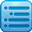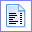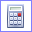Did you know that ...

Atlantis can automatically generate a Table Of Contents for your document. Use the Insert | Table Of Contents... menu command. Or press the corresponding toolbar button:Calculating in document window

In Atlantis you can perform calculations directly in the document window. For this type the required arithmetic expression in the document, select this expression, then press the Alt= keys or click the Calculatetoolbar button. If your expression is OK, Atlantis would place the computed result onto the clipboard so that you could paste it to this or any other document.

Your expressions for calculation could include integer numbers as well as real numbers both in floating point notation and scientific notation. For example:

732   -12.65   .65   34E87   14.3e-5

You can use the following arithmetic operators in your expressions:

 Operator Operation Sample expression + Addition 12.5+256 - Subtraction 112-7.6 * Multiplication 5*65 / or : Division 10/37.5:8

Your expressions can include the "percents" sign % and currency signs and abbreviations (\$, , £, ¥, USD). For example, you could use the following expressions in your documents:

\$15.50 + \$179.28
(1298 € + 9%) - 207.50 €

Tips:

• In most cases, you could calculate an expression even without selecting it. If the caret is within the required expression, or immediately after it, you could press the Alt= keys or click the Calculatetoolbar button. Atlantis would display a message suggesting to select the current expression automatically. After that you could press the Alt= keys or click the Calculatetoolbar button again to calculate the expression selected by Atlantis.
• You can quickly calculate the sum of multiple numbers (or multiple expressions). For this select the required numbers or expressions as a multiple selection. Then press the Alt= keys or click the Calculatetoolbar button. If all the selected numbers or expressions are OK, the sum would be placed onto the clipboard. In this way you could also calculate the sum of numbers selected a column block.